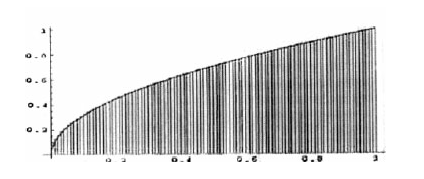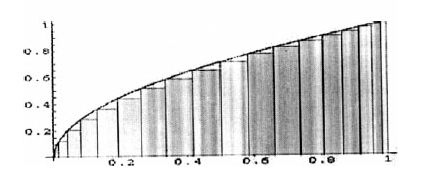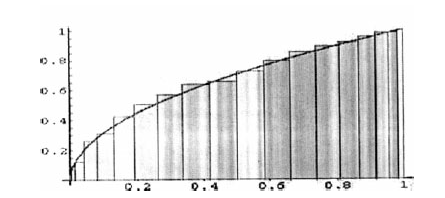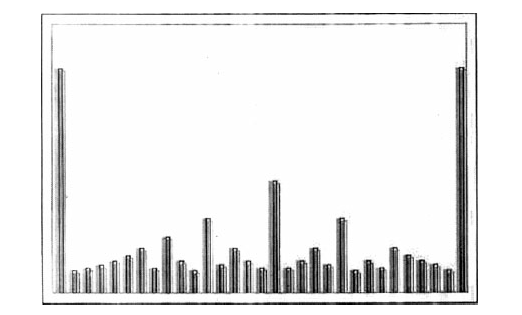## 黎曼（Georg Friedrich Bernhard Riemann，1826-1866）——生平与成就1849年黎曼获得学士学位后，回到哥廷根继续在高斯门下研读博士学位。1851年11月，黎曼向高斯提交了他的博士论文，标题为《复变函数一般理论的基础》。高斯在哥廷根的学术生涯此时已近尾声，他意识到他终于找到了一个配得上的继承者，并对黎曼的论文不吝溢美之词：（s,t为任意值，并满足$$x_{k-1}\leq s,t\leq x_k$$。）

• 如果x是有理数，且表达为最简分式a/b，则函数值为1/b。
• 如果x是无理数，函数值为0。• 函数的三角级数表示问题之历史
• 两个未知数的两个二次方程的解法
• 关于建立几何基础的假设

$$(\sum_{i,j=1}^nG_{ij}\Delta x_i\Delta y_i)^\frac{1}{2}$$

$$(x_1^2+x_2^2+x_3^2+...+x_n^2)^\frac{1}{2}$$

$$\frac{1}{1^s}+\frac{1}{2^s}+\frac{1}{3^s}+\frac{1}{4^s}+...+\frac{1}{n^s}+...$$

$$\zeta (s)=\sum_{n=1}^{\infty}\frac{1}{n^s}$$

$$\frac{1}{1^s}+\frac{1}{2^s}+\frac{1}{3^s}+\frac{1}{4^s}+...+\frac{1}{n^s}+...$$

$$(1+\frac{1}{2^1}+\frac{1}{2^2}+\frac{1}{2^3}+...+\frac{1}{2^k}+...)(1+\frac{1}{3^1}+\frac{1}{3^2}+\frac{1}{3^3}+...+\frac{1}{3^k}+...)(1+\frac{1}{5^1}+\frac{1}{5^2}+\frac{1}{5^3}+...+\frac{1}{5^k}+...)...$$

$$\zeta (s)=\prod_p\frac{1}{1-p^{-s}}$$

（如果f(x)=0，则x是函数f(x)的根。ζ(x)函数的根是实数，当且仅当Zeta函数的一个根是实部为$$\frac{1}{2}$$的复数。）做出这个关于Zeta函数的根的猜想后，黎曼总结道：

Zeta函数的根的值决定了π(x)和Li(x)之间差异的量级。“所有Zeta函数的根其实部都等于1/2”这个假设成为了“黎曼猜想”。

$$\pi (x)\sim Li(x)$$

$$Li(n)=\int_2^n \frac{dx}{ln(x)}$$

1900年，希尔伯特在国际数学家大会上的演讲中，将黎曼猜想作为他23个问题中的第8个。希尔伯特信心十足的认为黎曼猜想可以在10年左右时间内得到证明。黎曼猜想抵挡住了所有破解它的尝试，希尔伯特修正了这个预测。1943年他逝世前不久，有人问希尔伯特，如果他在500年后重生，他想问的第一个问题是什么。这位大数学家立即回答道，我会问：”黎曼猜想有人证明了吗？“